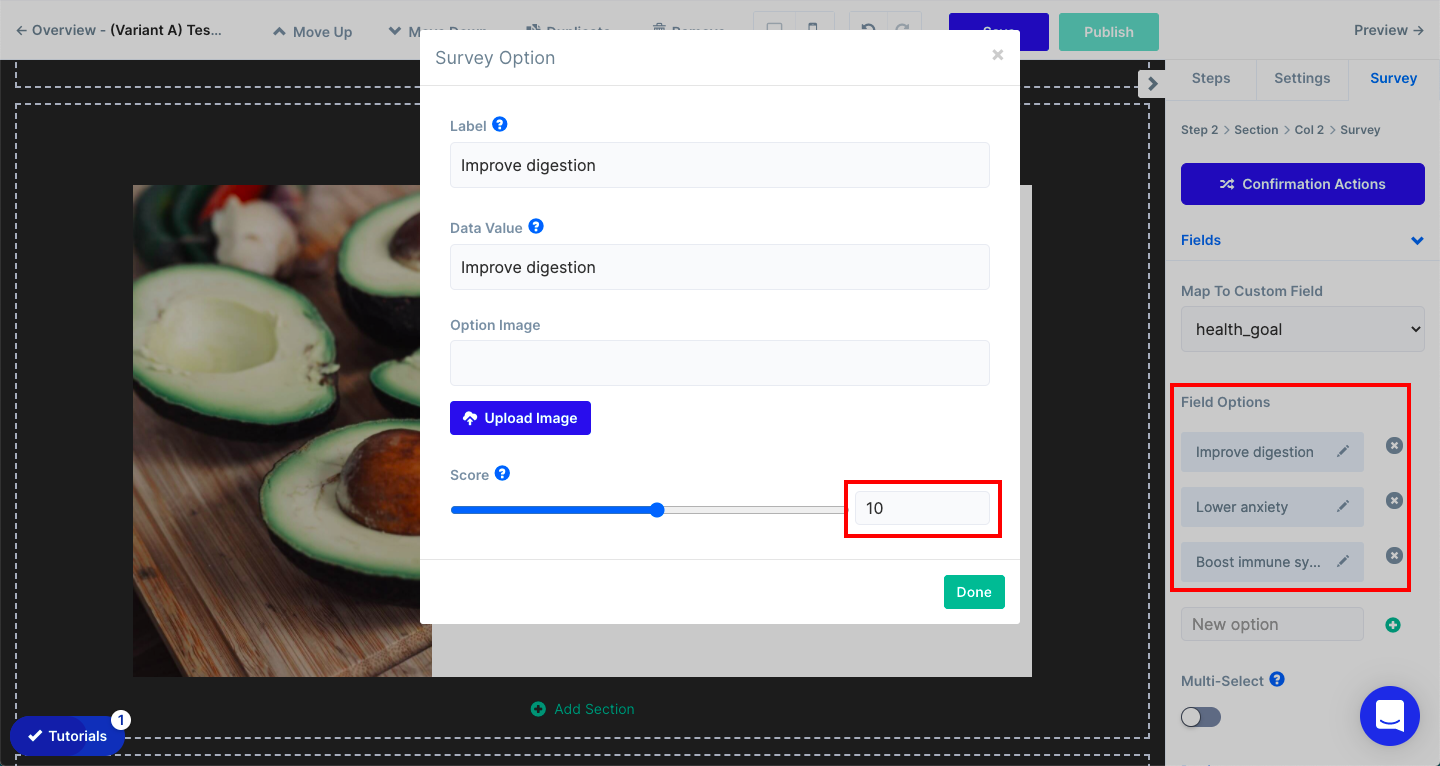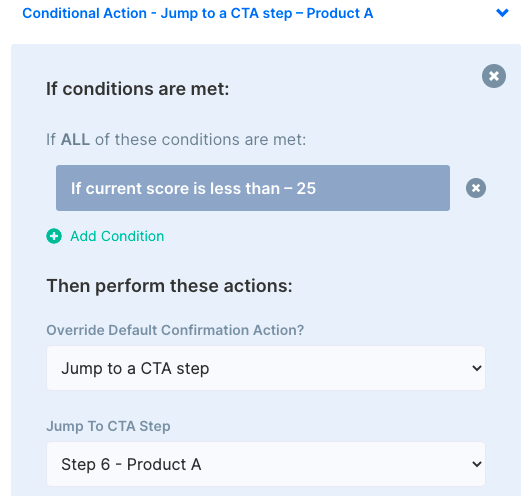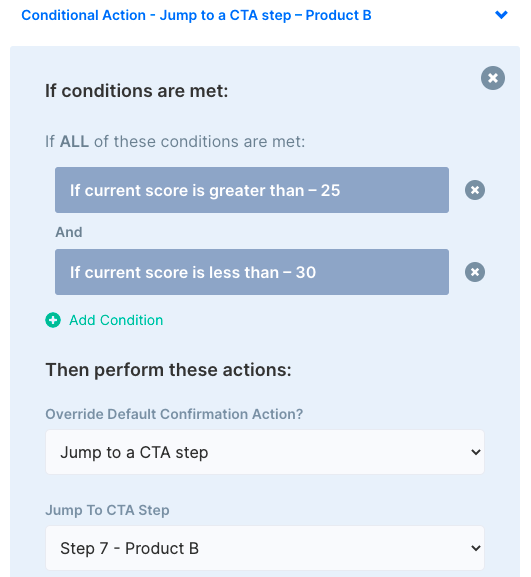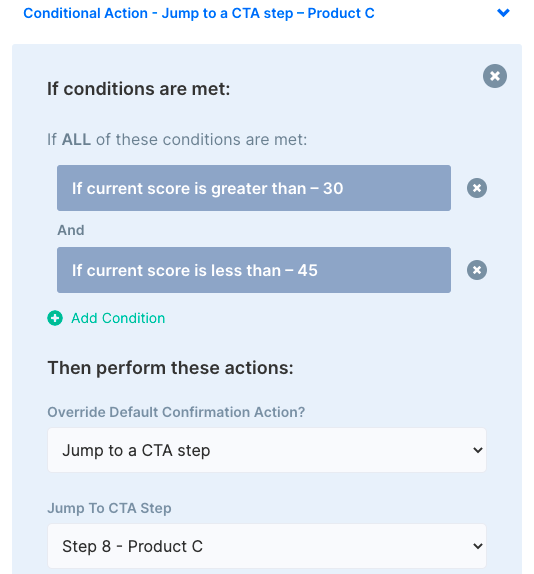# Creating a scored quiz

With our new scoring feature, displaying scored results for quizzes and surveys has never been so easy.

In this example, we’ll be using our Health Product Recommendation Quiz Template to display a scored result at the end of the quiz.

First, we’ll start by assigning the score value to each answer in the quiz. This can be done when editing the survey options:Once all the survey options on all the steps in the quiz have had a score assigned, all the possible score results can be calculated before setting the conditional actions to display the score in the different outcomes.

This is a good reference to make sure visitors see the correct outcome based on their score. In this case, we’ve assigned all options A are 5 points, options B are 10 points, and options C are 15 points. On a simple three-question/answer quiz, the results would be calculated like this:

 A A A 15 points A B A 20 points A C A 25 points A A B 20 points A B B 25 points A C B 30 points A A C 25 points A B C 30 points A C C 35 points B A A 20 points B B A 25 points B C A 30 points B A B 25 points B B B 30 points B C B 35 points B A C 30 points B B C 35 points B C C 40 points C A A 25 points C B A 30 points C C A 35 points C A B 30 points C B B 35 points C C B 40 points C A C 35 points C B C 40 points C C C 45 points

Here, where the minimum number of points is 15 and the maximum number is 45, we can set the conditional actions to send visitors to three different outcomes. We set the three tiers for the three outcomes like this:

• Outcome A: current score is less than 25 points
• Outcome B: current score is more than 25 and less than 30 points
• Outcome C: current score is more than 30 and less than 45 points

Now you’re ready to add these to the quiz form’s conditional actions and they’ll look like this:

• Outcome A: current score is less than 25 points• Outcome B: current score is more than 25 and less than 30 points• Outcome C: current score is more than 30 and less than 45 points• On the outcome steps you can include the merge tag ({CURRENT_SCORE}) to dynamically display the person’s score on their outcome page: